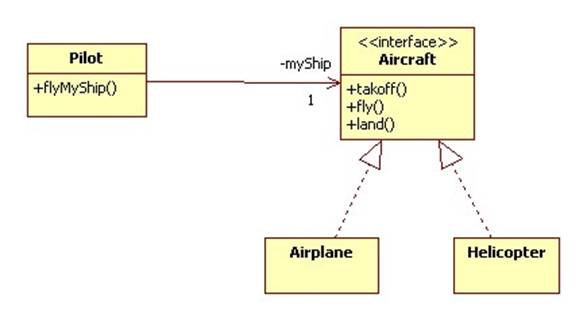# The Abstraction Principle

The Abstraction Principle is one of the most fundamental principles in engineering. It states:

The interface of a component should be independent of its implementation.

A component could be a hardware or software component.

The interface of a component is the user's view of the component, while the implementation is the developer's view. If a component is designed following the Abstraction Principle, then the user doesn't need to understand how a component works in order to use the component and the developer can modify the implementation without notifying the user.

## Example

The classical example of the Abstraction Principle is a car. We can regard the interface as the dashboard, pedals, and shifter. The implementation is the engine and transmission. Think about how difficult it would be if drivers needed to understand engines in order to drive and had to relearn to drive each time a mechanic replaced an engine part.

## Example (C)

The C Math library contains trigonometric functions for computing sines and cosines. We can identify the interfaces of these functions with their headers stored in math.h:

double sin(double x); /* computes sine of x radians */
double cos(double x); /* computes cosine of x radians */

The implementations are the associated algorithms (command blocks) contained in math.c:

double sin(double x) { ... }
double cos(double x) { ... }

Notice that scientists interested in computing sines and cosines can do so without having to understand the complex algorithms involved, while the developers of the math library can fine tune the algorithms without worrying about breaking the scientist's programs.

## Example (Java)

In Java we can explicitly declare interfaces and the classes that implement them. For example:

interface Aircraft {
void takeoff();
void fly();
void land();
}

class Airplane implements Aircraft {
public void takeoff() {
// algorithm for taking off in an airplane goes here
}
public void fly() {
// algorithm for flying an airplane goes here
}
public void land() {
// algorithm for landing an airplane goes here
}
}

class Helicopter implements Aircraft {
public void takeoff() {
// algorithm for taking off in a helicopter goes here
}
public void fly() {
// algorithm for flying a helicopter goes here
}
public void land() {
// algorithm for landing a helicopter goes here
}
}

A pilot can be independent of the type of aircraft he flies:

class Pilot {
private Aircraft myShip; // = Airplane or Helicopter
public void setMyShip(Aircraft ship) {
myShip = ship;
}
public void flyMyShip() {
myShip.takeoff();
myShip.fly();
myShip.land();
}
}

## Example (UML)

We can express the idea of the last example in UML:UML also allows us to express the same idea more generally using component diagrams:A component may consist of several classes that work together to implement an interface. For example, the Engine and Transmission classes may work together to implement the Dashboard interface in a computer simulation of a car.